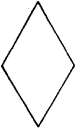# rhombus

(redirected from Diamond (geometry))
Also found in: Dictionary, Thesaurus.

## rhombus

an oblique-angled parallelogram having four equal sides

## Rhombus

a plane quadrangle with equal sides (Figure 1). It may be regarded as the special case of the parallelogram where two adjacent sides are equal, the diagonals are perpendicular,Figure 1

or a diagonal bisects the angles of the vertices that it joins. A rhombus with right angles is called a square.

## rhombus

[′räm·bəs]
(mathematics)
A parallelogram with all sides equal. Also known as rhomb.
Site: Follow: Share:
Open / Close